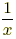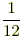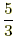index: click on a letter A B C D E F G H I J K L M N O P Q R S T U V W X Y Z A to Z index index: subject areas numbers & symbols sets, logic, proofs geometry algebra trigonometry advanced algebra & pre-calculus calculus advanced topics probability & statistics real world applications multimedia entrieswww.mathwords.com about mathwords website feedback

 Multiplicative Inverse of a Number Reciprocal The reciprocal of x is. In other words, a reciprocal is a fraction flipped upside down. Multiplicative inverse means the same thing as reciprocal. For example, the multiplicative inverse (reciprocal) of 12 isand the multiplicative inverse (reciprocal) ofis.   Note: The product of a number and its multiplicative inverse is 1. Observe that·= 1.     See also Inverse